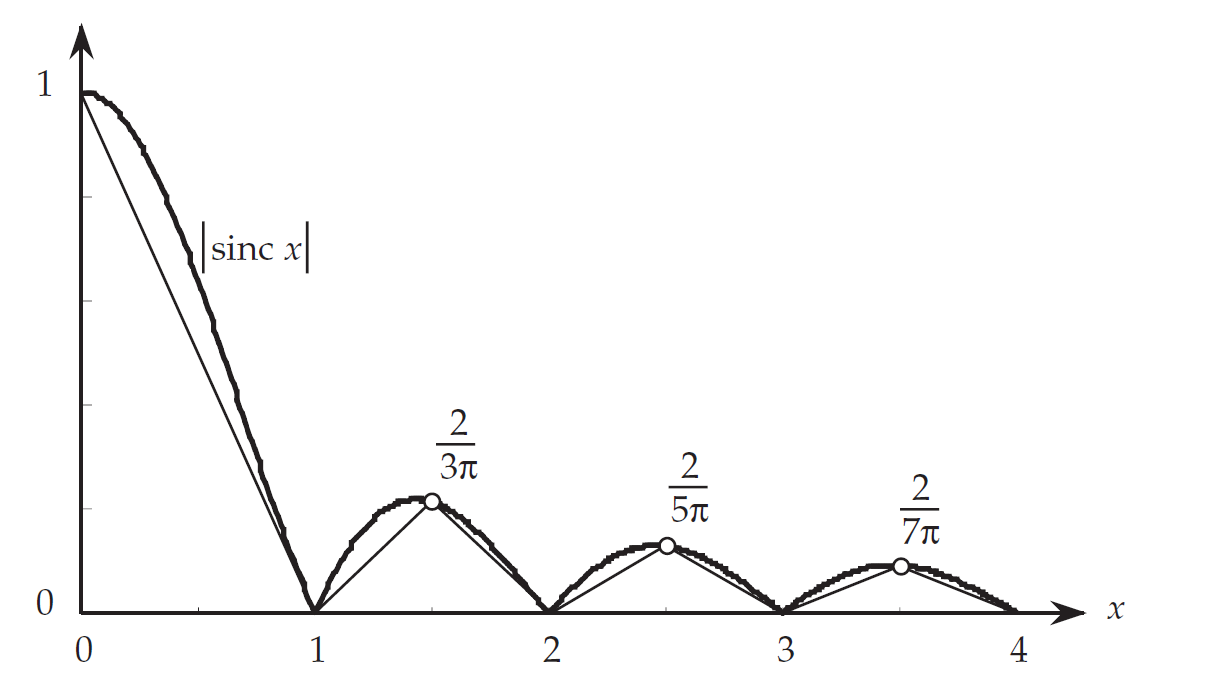# $\mathrm{sinc}(x)$在$[0,+\infty)$不绝对可积的证明

sinc 函数的定义是：

\begin{equation} \mathrm{sinc} x = \frac{\sin{\pi x}}{\pi x} \end{equation}

\begin{equation} \int_{-\infty}^{\infty}|\mathrm{sinc}(x)| dx = 2 \int_{0}^{\infty} \frac{|\sin{\pi x}|}{|\pi x|} dx \end{equation}\begin{equation} \lVert\mathrm{sinc}\rVert_1>2\left(\frac{1}{2}+\sum_{k=1}^{\infty}I_k\right)=1+\frac{2}{\pi}\sum_{k=1}^{\infty}\frac{1}{2k+1} \end{equation}

## 展开本分类索引